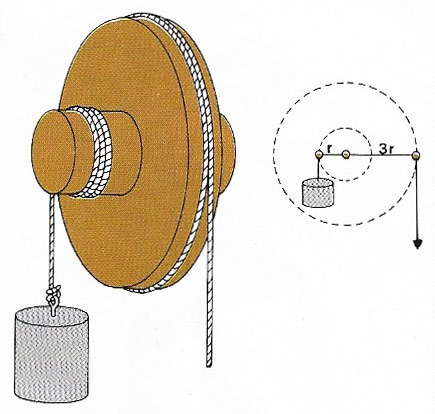A# mechanical advantageMechanical advantage is the ratio of force exerted by a machine to the force exerted on it. In this wheel-and-axel pulley the radius of the wheel is three times that of the axel. Theoretically, then, the mechanical advantage is three, that is, a downwards force on the rope should result in an upwards force on the weight three times greater. In practice, however, friction always lowers mechanical advantage.

Mechanical advantage, also called force ratio, is the ratio of the output force (load) of a machine to the input force (effort). In the case of an ideal (frictionless and weightless) machine, mechanical advantage = velocity ratio.